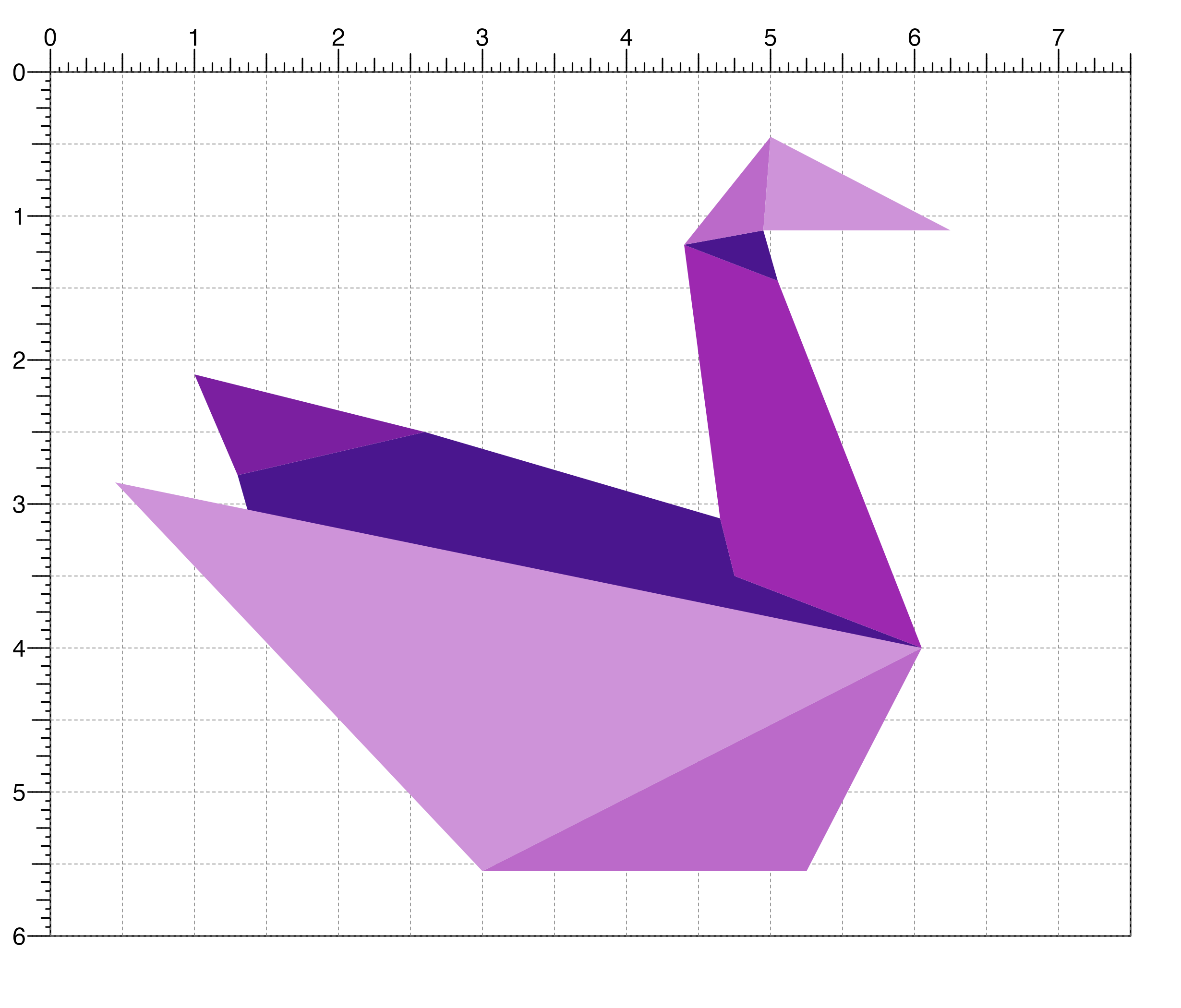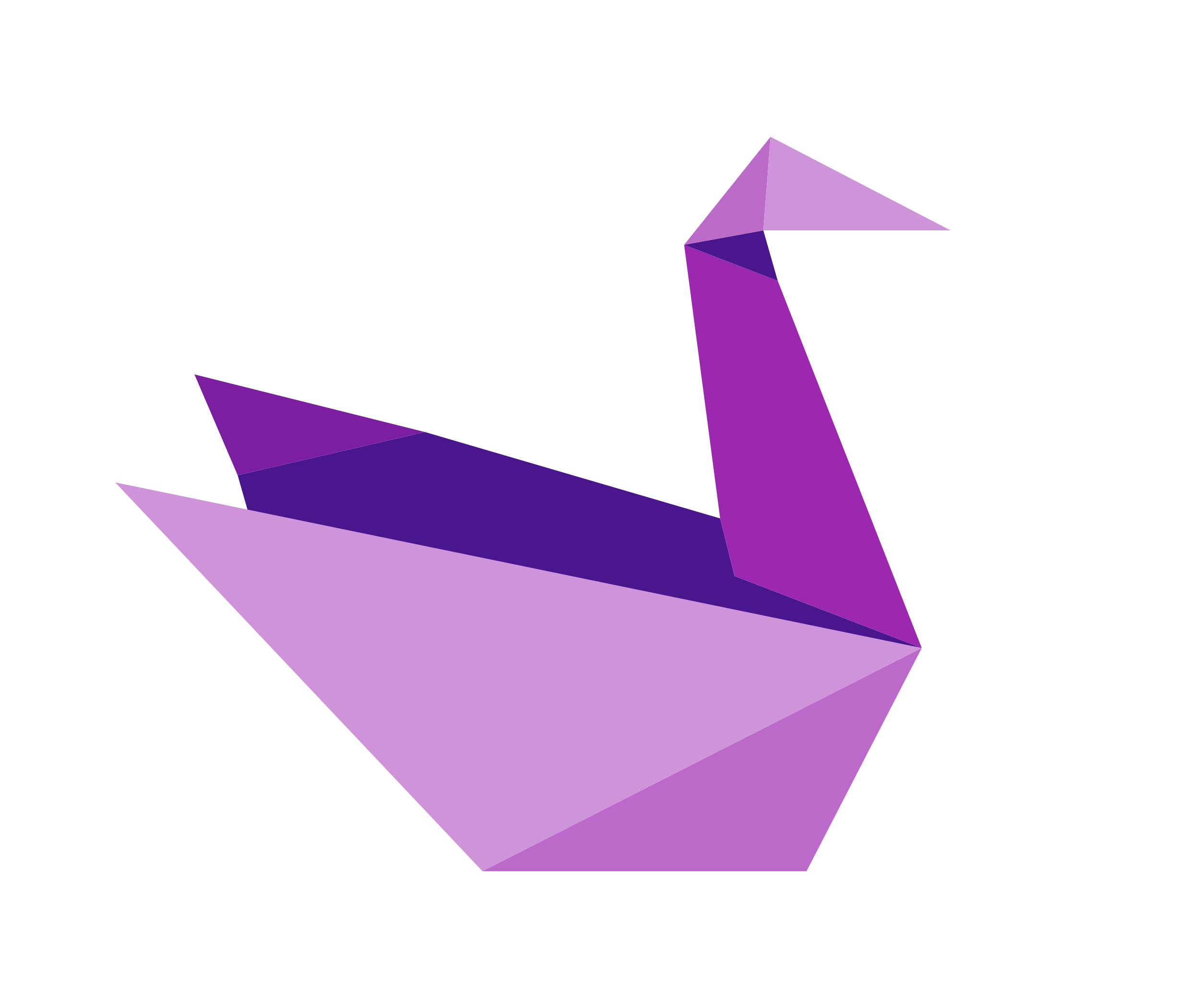Plot a polygon within a plotgardener layout

plotPolygon(
x,
y,
default.units = "inches",
linecolor = "black",
lwd = 1,
lty = 1,
fill = NA,
alpha = 1,
id = NULL,
id.lengths = NULL,
params = NULL,
...
)

## Arguments

x

A numeric vector or unit object specifying polygon vertex x-locations.

y

A numeric vector, unit object, or a character vector of values containing a "b" combined with a numeric value specifying polygon vertex y-locations. The character vector will place polygon vertex y-locations relative to the bottom of the most recently plotted plot according to the units of the plotgardener page.

default.units

A string indicating the default units to use if x or y are only given as numeric vectors. Default value is default.units = "inches".

linecolor

A character value specifying polygon line color. Default value is linecolor = "black".

lwd

A numeric specifying polygon line width. Default value is lwd = 1.

lty

A numeric specifying polygon line type. Default value is lty = 1.

fill

A character value specifying polygon fill color. Default value is fill = NA.

alpha

Numeric value specifying color transparency. Default value is alpha = 1.

id

A numeric vector used to separate locations in x and y into multiple polygons. All locations with the same id belong to the same polygon.

id.lengths

A numeric vector used to separate locations in x and y into multiple polygons. Specifies consecutive blocks of locations which make up separate polygons.

params

An optional pgParams object containing relevant function parameters.

...

Additional grid graphical parameters. See gpar.

## Value

Returns a polygon object containing relevant placement and grob information.

## Examples

## Create a page
pageCreate(width = 7.5, height = 6, default.units = "inches")

## Plot complex polygons one at a time
plotPolygon(
x = c(2.6, 4.65, 4.75, 6.05, 1.4, 1.3),
y = c(2.5, 3.1, 3.5, 4, 3.15, 2.8),
fill = "#4a168e", linecolor = NA
)
#> polygon[polygon1]

plotPolygon(
x = c(4.65, 4.75, 6.05, 5.05, 4.4),
y = c(3.1, 3.5, 4, 1.45, 1.2),
fill = "#9d28b0", linecolor = NA
)
#> polygon[polygon2]

## Plot multiple triangles with different id's and colors
plotPolygon(
x = c(
0.45, 6.05, 3, 3, 6.05, 5.25, 4.4, 5.05, 4.95,
1.3, 2.6, 1, 4.4, 4.95, 5, 4.95, 5, 6.25
),
y = c(
2.85, 4, 5.55, 5.55, 4, 5.55, 1.2, 1.45, 1.1,
2.8, 2.5, 2.1, 1.2, 1.1, 0.45, 1.1, 0.45, 1.1
),
id = c(1, 1, 1, 2, 2, 2, 3, 3, 3, 4, 4, 4, 5, 5, 5, 6, 6, 6),
fill = c(
"#ce93d9", "#bb6ac9", "#4a168e",
"#7b1fa0", "#bb6ac9", "#ce93d9"
),
linecolor = NA
)
#> polygon[polygon2]## Hide page guides
pageGuideHide()